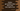# Python program to get random values from a list, dictionary, tuple or set## Introduction :

sample() method is defined in random module of Python. It is used to get a random length list of unique elements. We can provide the length of the final list and it will pick some random elements from the given sequence or set. Note that elements of the provided list need not be unique. If it contains any duplicate elements, that the final result may pick duplicates. In this post, we will learn how sample method works with different examples.

### Syntax of sample :

This method is defined as below :

``random.sample(data, k)``

Here, data is the given sequence or set and k is the final length of list that we want to pick from data.

It raises one ValueError if the size of k is more than the size of data .

### Find Random sample from a range of integers :

Let’s start with a simple example. To find random list from a range of integers, we can use range . The advantage of using range is that it is more memory efficient than list or tuple .

``````import random
print(random.sample(range(1000),5))``````

If you run the above program, it will print five random numbers each time you execute. It returns one list. You will get output as like below :

``````[804, 188, 683, 921, 626]
[557, 589, 85, 851, 278]
[371, 443, 803, 753, 263]``````

Note that we need to import the random module to use this method.

### Errors :

random.sample() is throwing a ValueError() : This is a common problem with sample . If the value of k is larger than the total size of data or if its value is negative, it will throw ValueError . For example :

``````import random
print(random.sample(range(3),5))``````

If you run this program, it will give one ValueError as like below :

``````Traceback (most recent call last):
File "example.py", line 3, in <module>
print(random.sample(range(3),5))
File "/usr/local/Cellar/python/3.7.5/Frameworks/Python.framework/Versions/3.7/lib/python3.7/random.py", line 321, in sample
raise ValueError("Sample larger than population or is negative")
ValueError: Sample larger than population or is negative``````

### TypeError:

sample throws TypeError if any of the parameter is missing. It will say that missing 1 required positional argument . For example :

``````import random
print(random.sample(range(3)))``````

This program will throw TypeError :

``````Traceback (most recent call last):
File "example.py", line 3, in <module>
print(random.sample(4))
TypeError: sample() missing 1 required positional argument: 'k'``````

### Example to get one random list from a Python dictionary using sample:

We have learned how to use random() with one simple example. Let’s try it now with a dictionary. The problem is that you can’t use dictionary directly with random() because it works only with a sequence or set and dictionary is not a sequence. Instead, we can use dictionary.items() method that returns a list of all dictionary key value pairs. It doesn’t take any parameters.

Let’s take a look at the example below :

``````import random
data = {
'one': 1,
'two': 2,
'three': 3,
'four': 4,
'five': 5,
'six': 6,
'seven': 7,
'eight': 8,
'nine': 9,
'ten': 10
}
print(random.sample(data.items(), 3))``````

It will print one output with three items of data as like below :

``[('seven', 7), ('ten', 10), ('three', 3)]``

### How to use python random.sample() with tuple :

We can use sample() directly with a tuple. For example :

``````import random
dataTuple = (1, 2, 3, 'four', 'five' , 6)
print(random.sample(dataTuple,2))``````

It works as like the other examples. Each time you run this program, it will print two values from the tuple.

``````['five', 3]
[1, 3]``````

### Using python random.sample() with a set :

As I have mentioned before, sample() works with python set as well. It works in a similar manner :

``````import random
dataSet = [1,2,3,4,5]
print(random.sample(dataSet,2))``````

It will print similar output.

### Conclusion :

Python random.sample() is a useful method to get a list of random data from a list or set. It helps us to get a random data list easily. In this post, we have seen how to use sample() with different data types. If you want only a single random item, you can use random.choice() method.